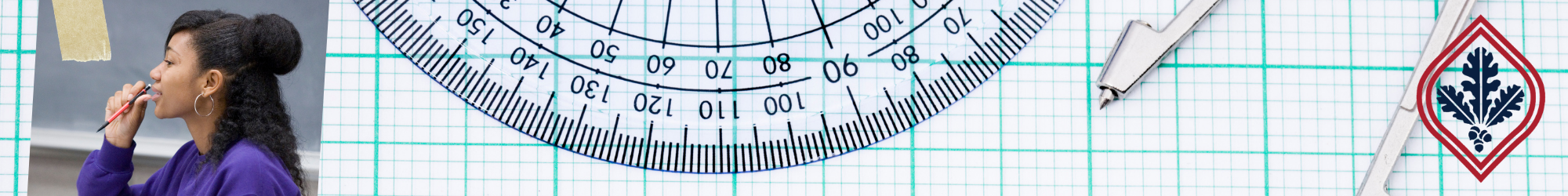# Assessment

Starting Fall 2022, pre-transfer level Math and English courses are no longer offered at SRJC. Learn More.## Math Sequence Chart

The math sequence chart shown below is intended to help you understand your math placement. Your starting point depends on your math placement result, educational goal, and prior experience in mathematics. Before enrolling in a math class, please meet with a counselor.

Math Level Science, Technology,
Engineering, & Mathematics
(STEM)
(without transfer)
Four Levels Below Transfer College Skills 371 (3.5 units)
General Arithmetic
College Skills 371 (3.5 units)
General Arithmetic
College Skills 371 (3.5 units)
General Arithmetic
College Skills 371 (3.5 units)
General Arithmetic
Three Levels Below Transfer College Skills 372 (3.5 units)
Pre-Algebra
OR
College Skills 373 (3.5 units)
Arithmetic Foundation
(CSKLS 373 is a combination of CSKLS 371 & 372)
College Skills 372 (3.5 units)
Pre-Algebra
OR
College Skills 373 (3.5 units)
Arithmetic Foundation
(CSKLS 373 is a combination of CSKLS 371 & 372)

College Skills 372 (3.5 units)
Pre-Algebra
OR
College Skills 373 (3.5 units)
Arithmetic Foundation
(CSKLS 373 is a combination of CSKLS 371 & 372)

College Skills 372 (3.5 units)
Pre-Algebra
OR
College Skills 373 (3.5 units)
Arithmetic Foundation
(CSKLS 373 is a combination of CSKLS 371 & 372)
Two Levels Below Transfer Math 150 (4 units)
Elementary Algebra
Math 150 (4 units)
Elementary Algebra
Math 150 (4 units)
Elementary Algebra
Math 150 (4 units)
Elementary Algebra
One Level Below Transfer Math 154 (8 units)
Elementary & Intermediate Algebra
(Math 154 is a combination of Math 150 & 156)
OR
Math 156 (5 units)
Intermediate Algebra for BSTEM
Math 154 (8 units)
Elementary & Intermediate Algebra
(Math 154 is a combination of Math 150 & 156)
OR
Math 156 (5 units)
Intermediate Algebra for BSTEM
Math 161 (4 units)
Statistics & Liberal Arts Math Prep
Math 101 (3 units)
Math for AA/AS Degree
OR
Any math course at this level: 154, 156, 161, or higher
Transfer Level Math Math 27 (6 units)
Precalculus Algebra & Trigonometry
(Math 27 is a combination of Math 25 & Math 58)
OR
Math 25 (4 units)
Precalculus Algebra
May be required to take concurrent support, Math 200 (2 units)*
AND
Math 58 (3 units)
Precalculus Trigonometry
(Math 25 is the prerequisite to Math 58)
Math 9 (3 units)
Finite Mathematics
May be required to take concurrent support, Math 200 (2 units)*
OR
Math 15 (4 units)
Elementary Statistics
May be required to take concurrent support, Math 215 (2 units)**
OR
Math 16 (4 units)
Intro to Math Analysis
Math 10 (3 units)
Nature of Mathematics
OR
Math 15 (4 units)
Elementary Statistics
May be required to take concurrent support, Math 215 (2 units)**
Math 10 (3 units)
Nature of Mathematics
OR
Math 15 (4 units)
Elementary Statistics
May be required to take concurrent support, Math 215 (2 units)**
Higher Level Math Math 1A (5 units)
Calculus, First Course
OR
Other higher-level math course

*Math 200 (2 units) B-STEM Concurrent Support, may be required with your Math 9 & Math 25 course. Please refer to the Math Placement Calculations to learn more.

**Math 215 (2 units) Elementary Statistics Concurrent Support, is required for students who did not pass Algebra 2 or Integrated Math 3 and is recommended for students with a GPA less than a 2.7.